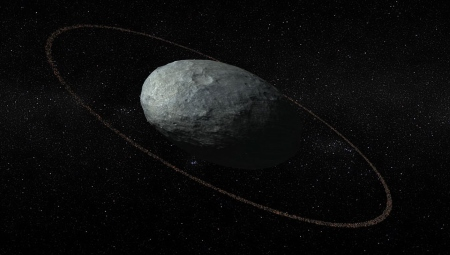# Ellipsoid geometry and Haumea

To first approximation, Earth is a sphere. A more accurate description is that the earth is an oblate spheroid, the polar axis being a little shorter than the equatorial diameter. See details here. Other planets are also oblate spheroids as well. Jupiter is further from spherical than the earth is more oblate.

The general equation for the surface of an ellipsoid isAn ellipsoid has three semi-axes: ab, and c. For a sphere, all three are equal to the radius. For a spheroid, two are equal. In an oblate spheroid, like Earth and Jupiter, abc. For a prolate spheroid like Saturn’s moon Enceladus, abc.The dwarf planet Haumea is far from spherical. It’s not even a spheroid. It’s a general (tri-axial) ellipsoid, with a, b, and c being quite distinct. It’s three semi-axes are approximately 1000, 750, and 500 kilometers. The dimensions are not known very accurately. The image above is an artists conception of Haumea and its ring, via Astronomy Picture of the Day.

This post explains how to compute the volume and surface area of an ellipsoid. See that post for details. Here we’ll just copy the Python code from that post.

```    from math import sin, cos, acos, sqrt, pi
from scipy.special import ellipkinc, ellipeinc

def volume(a, b, c):
return 4*pi*a*b*c/3

def area(a, b, c):
phi = acos(c/a)
k = a*sqrt(b**2 - c**2)/(b*sqrt(a**2 - c**2))
E = ellipeinc(phi, k)
F = ellipkinc(phi, k)
elliptic = E*sin(phi)**2 + F*cos(phi)**2
return 2.0*pi*c**2 + 2*pi*a*b*elliptic/sin(phi)

a, b, c = 1000, 750, 500

print(volume(a, b, c))
print(area(a, b, c))
```

Related post: Planets evenly spaced on a log scale

## One thought on “Ellipsoid geometry and Haumea”

1. Richard Holmes

I was surprised by the statement that Enceladus is a prolate spheroid. I found the following, which seems to say it is triaxial, like (but less extremely than) Haumea, but closer to an oblate spheroid with the polar semimajor axis less than either of the equatorial semimajor axes: “Limb profiles from Cassini images show that Enceladus is well represented by a triaxial ellipsoid whose principal axes are a = 256.6 ± 0.3 km, b = 251.4 ± 0.2 km, and c = 248.3 ± 0.2 km” (c being the polar semimajor axis; see figure 1) at https://agupubs.onlinelibrary.wiley.com/doi/10.1002/jgre.20122 .